# JEE Main Carboxylic Acid Previous Year Questions with Solutions

Carboxylic Acid previous year questions and solutions are given in this article. Carboxylic acid is an organic compound containing a carboxyl group (COOH) attached to an alkyl or aryl group. The general formula is CnH2n+1COOH. In these acids, three of the four bonds of the carboxyl carbon atom are with two oxygen atoms. They have high boiling points as compared to aldehydes and ketones of comparable masses because of intermolecular hydrogen bonding. As the number of carbon atoms in carboxylic acids increases, their solubility decreases due to an increase in the length of the hydrophobic group attached to the carboxyl group. This article helps students to revise the previous years’ questions and score higher rank in the JEE exam.

As far as the JEE exam is concerned, Carboxylic Acid is an important topic. The questions given here give you an idea about what type of questions to expect from this topic. BYJU’S provides accurate solutions created by our subject experts. Students can easily download these solutions in PDF format for free.

## JEE Main Previous Year Solved Questions on Carboxylic Acid

1. The correct decreasing order for acid strength is

(1) FCH2COOH > NCCH2COOH > NO2CH2COOH > ClCH2COOH

(2) CNCH2COOH > O2NCH2COOH > FCH2COOH > ClCH2COOH

(3) NO2CH2COOH > NCCH2COOH > FCH2COOH > ClCH2COOH

(4) NO2CH2COOH > FCH2COOH > CNCH2COOH > ClCH2COOH

Solution:

Order of electron-withdrawing effect is -NO2 > -CN > -F > -Cl. So the acid strength order is NO2CH2COOH > NCCH2COOH > FCH2COOH > ClCH2COOH.

Hence option (3) is the answer.

2. In the chemical reactions,The compounds ‘A’ and ‘B’ are respectively:

(1) Nitrobenzene and chlorobenzene

(2) Nitrobenzene and fluorobenzene

(3) Phenol and benzene

(4) Benzene diazonium chloride and fluorobenzene

Solution: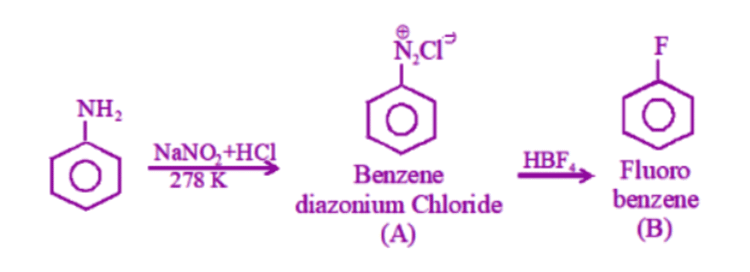Hence option (4) is the answer.

3. On heating an aliphatic primary amine with chloroform & ethanolic potassium hydroxide the organic compound formed is

(1) An alkyl cyanide

(2) An alkyl isocyanide

(3) an alkanol

(4) an alkane diol

Solution:

On heating, an aliphatic primary amine with chloroform and ethanolic potassium hydroxide the organic compound formed is an alkyl isocyanide. This reaction is called the Carbylamine reaction.

R-NH2 + CHCl3 → R-NC

Hence option (2) is the answer.

4. In the chemical reactions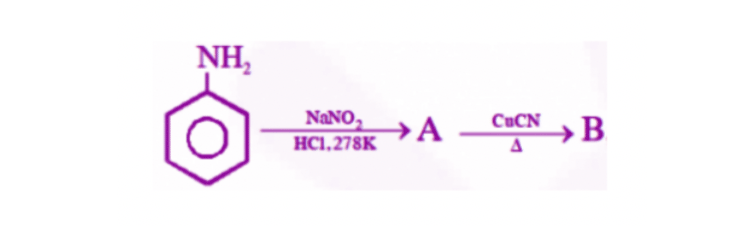The compounds ‘A’ and ‘B’ are respectively:

(1) Fluorobenzene and phenol

(2) Benzene diazonium chloride and benzonitrile

(3) Nitrobenzene and chlorobenzene

(4) Phenol and bromobenzene

Solution: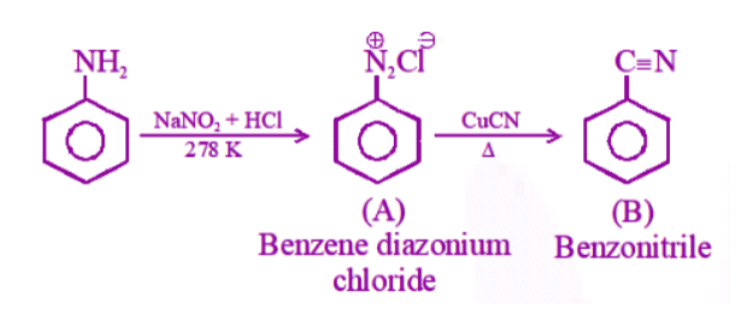Compound A is Benzene diazonium chloride.

Compound B is benzonitrile.

Hence option (2) is the answer.

5. When CH2 = CH- COOH is reduced with LiAlH4, the compound obtained will be

(1) CH2 = CH-CH2OH

(2) CH3-CH2 -CHOH

(3) CH3-CH-CHO

(4) CH3-CH2-COOH

Solution:

LiAlH4 can reduce the COOH group and not the double bond.

CH2 = CH-COOH is reduced to give CH2 = CH-CH2OH

Hence option (1) is the answer.

6. Consider the acidity of the carboxylic acids: Most acidic is

(1) PhCOOH

(2) o – N02C6H4C00H

(3) p – N02C6H4C00H

(4) m – N02C6H4C00H

Solution:

Due to the ortho effect, the acidity of ortho nitrobenzoic acid is greater when compared to other given compounds.

Hence option (2) is the answer.

7. Among the following acids which have the lowest pKa value?

(1) CH3COOH

(2) HCOOH

(3) (CH3)2COOH

(4) CH3CH2COOH

Solution:

HCOOH is the most acidic. So it has the lowest pKa value.

Hence option (2) is the answer.

8. The correct order of increasing acid strength of the compounds is

(i) CH3COOH

(ii) MeOCH2COOH

(iii) CF3COOH

(iv) (Me)2 COOH

(1) ii < iv < i < iii

(2) iv < i < iii < ii

(3) iv < i < ii < iii

(4) i < iv < iii < ii

Solution:

The presence of the electron-withdrawing group increases acidic strength.

The correct order of increasing acid strength is iv < i < ii < iii.

Hence option (3) is the answer.

9. A liquid was mixed with ethanol and a drop of concentrated H2SO4 was added. A compound with a fruity smell was formed. The liquid was

(1) CH3OH

(2) HCHO

(3) CH3COCH3

(4) CH3COOH

Solution:

The fruity smell occurs due to the formation of ester.

CH3COOH + CH3CH2OH → CH3COOC2H5

Hence option (4) is the answer.

10. p-cresol reacts with chloroform in alkaline medium to give the compound A which adds hydrogen cyanide to form compound B. The latter on acidic hydrolysis gives chiral carboxylic acid. The structure of the carboxylic acid is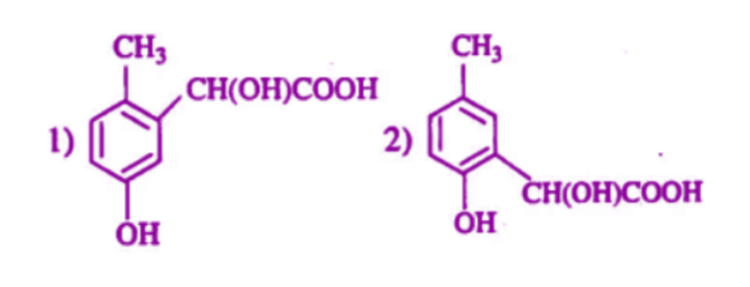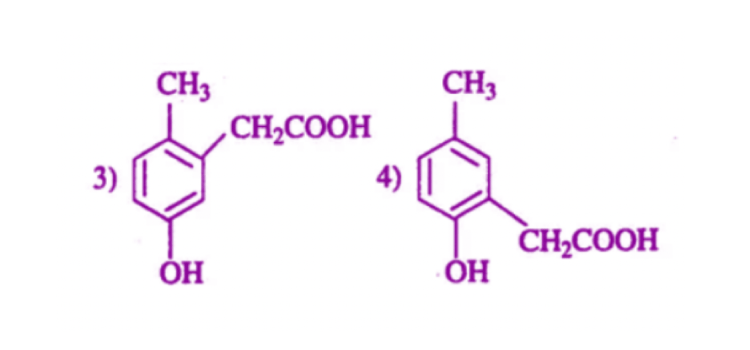Solution:

The reaction of phenol derivative with chloroform in alkaline medium is Reimer-Tiemann reaction. The addition of HCN at aldehyde group will result in cyanohydrin formation.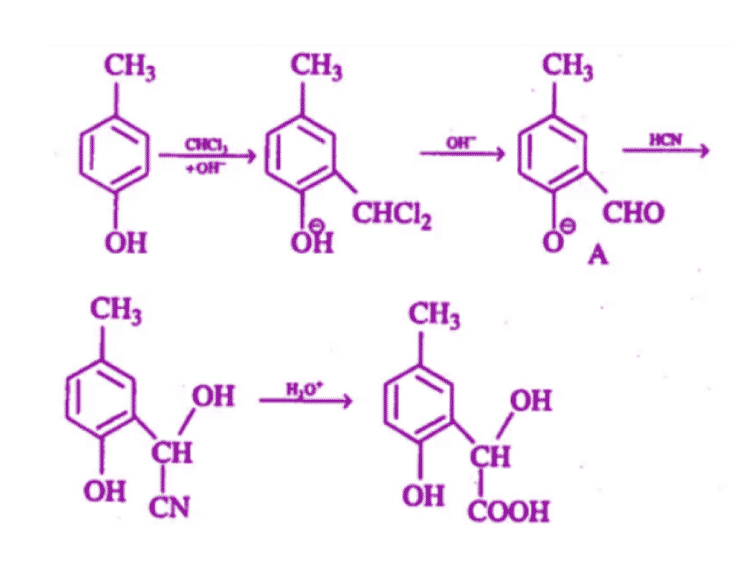Hence option (2) is the answer.

11. The strongest acid amongst the following compounds is

(1) CH3CH2CH(Cl)COOH

(2) ClCH2CH2CH2 COOH

(3) CH3COOH

(4) HCOOH

Solution:

Due to -I effect of Cl, CH3CH2CH(Cl)COOH is the strongest acid.

Hence option (1) is the answer.

12. The general formula CnH2nO2 could be for open chain

(1) diketones

(2) carboxylic acids

(3) diols

(4) dialdehydes.

Solution:

The general formula CnH2nO2 could be for open chain carboxylic acids.

Hence option (2) is the answer.

13. What is B?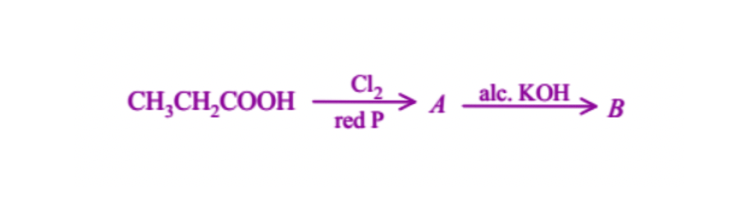(1) CH3CH2COCl

(2) CH3CH2CHO

(3) CH2 = CHCOOH

(4) ClCH2CH2COOH

Solution: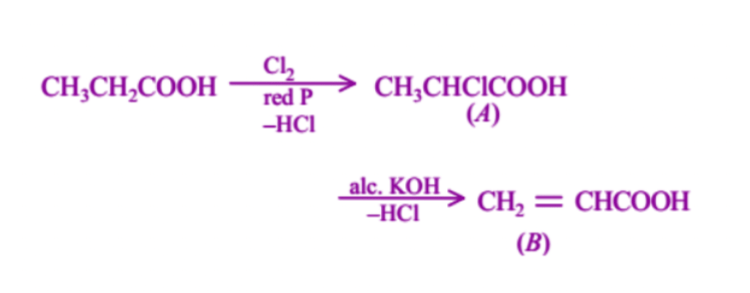Hence option (3) is the answer.

14. In the reaction,C is:

(1) Ethylene

(2) Acetyl chloride

(3) Acetaldehyde

(4) Acetylene.

Solution:

The reduction of acetic acid gives CH3-CH2-OH (A). The reaction of CH3-CH2-OH with PCl5 will give CH3-CH2-Cl (B). when it is treated with alcoholic KOH, the product obtained is CH2=CH2.

Hence option (1) is the answer.

15. In the presence of a small amount of phosphorous, aliphatic carboxylic acids react with chlorine or bromine to yield a compound in which α– hydrogen has been replaced by halogen. This reaction is known as :

(1) Etard reaction

(2) Hell -Volhard – Zelinsky reaction

(3) Wolff -Kishner reaction

(4) Rosenmund reaction

Solution:

Hell -Volhard – Zelinsky reaction is the reaction in which aliphatic carboxylic acids react with chlorine or bromine to yield a compound in which α– hydrogen has been replaced by halogen, in the presence of a small amount of phosphorus.

Hence option (2) is the answer.